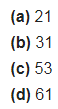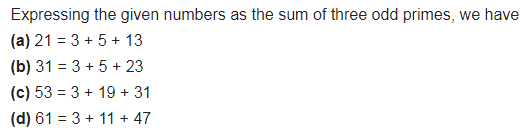# Express each of the following numbers as the sum of three odd primes: (a) 21

Express each of the following numbers as the sum of three odd primes: